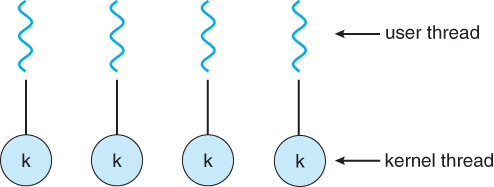387 views

User level threads are threads that are visible to the programmer and are unknown to the kernel. The operating system kernel supports and manages kernel level threads. Three different types of models relate user and kernel level threads.

Which of the following statements is/are true?

1.
1. The Many – to – one model maps many user threads to one kernel thread
2. The one – to – one model maps one user thread to one kernel thread
3. The many – to – many model maps many user threads to smaller or equal kernel threads
2.
1. Many – to – one model maps many kernel threads to one user thread
2. One – to – one model maps one kernel thread to one user thread
3. Many – to – many model maps many kernel threads to smaller or equal user threads
1. (a) is true; (b) is false
2. (a) is false; (b) is true
3. Both (a) and (b) are true
4. Both (a) and (b) are false

A is true, B is false.

For detailed explanation  please refer to Galvin book Section 4.2

### 1 comment

I don't have book can u pls post a screenshot?

Option A

1 Many-To-One Model

• In the many-to-one model, many user-level threads are all mapped onto a single kernel thread.
• Thread management is handled by the thread library in user space, which is very efficient.
• However, if a blocking system call is made, then the entire process blocks, even if the other user threads would otherwise be able to continue.
• Because a single kernel thread can operate only on a single CPU, the many-to-one model does not allow individual processes to be split across multiple CPUs.
• Green threads for Solaris and GNU Portable Threads implement the many-to-one model in the past, but few systems continue to do so today.Figure 1 - Many-to-one model

.2 One-To-One Model

• The one-to-one model creates a separate kernel thread to handle each user thread.
• One-to-one model overcomes the problems listed above involving blocking system calls and the splitting of processes across multiple CPUs.
• However the overhead of managing the one-to-one model is more significant, involving more overhead and slowing down the system.
• Most implementations of this model place a limit on how many threads can be created.
• Linux and Windows from 95 to XP implement the one-to-one model for threads.Figure 2- One-to-one model

.3 Many-To-Many Model

• The many-to-many model multiplexes any number of user threads onto an equal or smaller number of kernel threads, combining the best features of the one-to-one and many-to-one models.
• Users have no restrictions on the number of threads created.
• Blocking kernel system calls do not block the entire process.
• Processes can be split across multiple processors.
• Individual processes may be allocated variable numbers of kernel threads, depending on the number of CPUs present and other factors.Figure 3- Many-to-many model

• One popular variation of the many-to-many model is the two-tier model, which allows either many-to-many or one-to-one operation.
• IRIX, HP-UX, and Tru64 UNIX use the two-tier model, as did Solaris prior to Solaris 9.Figure 4. - Two-level model

1 vote